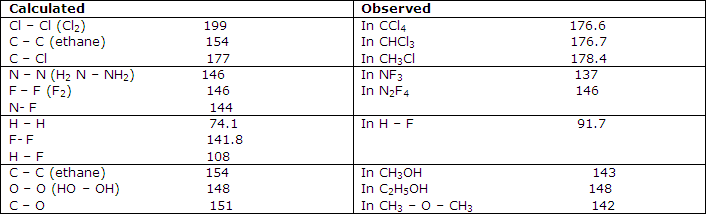What is covalent radii? Explain its calculation.

Average covalent radii can be assigned on the basis of molecular structures.

The accumulation of structural data by spectroscopic studies and both electron and x-ray diffraction studies allows one to investigate the possibility fo assigning a covalent bound molecule, i.e. of assigning a covalent radius to each atom. One begins by assigning half the length of a homonuclear bond as the covalent radius of the atoms forming the bond. Thus, from the equilibrium bond length of Cl2 of 199 pm, one obtains the value of 100 pm for the covalent radius of chlorine. From the carbon-carbon distance of 154 pm in ethane, for example, one obtains a value of 77 pm for the covalent radius of carbon and so forth. To proceed, one must now establish the extent to which the length of covalent bonds can be treated in terms of the sums of such covalent radii.

More extensive treatments of this type show that the bond lengths of many bonds are given within a few picometers by the sum of assigned atomic covalent radii. This suggests that covalent bonds have lengths sufficiently independent of factors other than the fixed radii for there to be some value in assigning radii to the bonded nuclei.Further comparisons of these values with experimental results indicate, as shown in fact by some of the examples of table 1, that serious discrepancies can occur between simply predicted covalent-bond lengths and those observed. The C-F bond, for example, is calculated from the data of table 1 to have a length of 146 pm, whereas microwave spectral results forCH3F give it as 138.5 pm and electron-diffraction results for CF4 give 132 pm.

Such discrepancies led V. Schomaker and D. P. Stevenson to suggest that a bond length calculated from covalent radii must be adjusted for the difference in electronegativity of the bonded atoms. They suggested the relation:

rAB = rA + rB - 90 (xA - xB) r in pm

Some but not all, the interesting violations of simple covalent radii additivity are removed by this empirical expression. In other cases the Stevenson-Schomaker correction makes the agreement with the observed length pooper than that obtained by a simple addition of the covalent radii. Although a number of factors must be operating to affect the length of a bond between a pair of nuclei in any given molecule, the covalent radii of table 2 are often of value in estimating this bond length.

Covalent radii for atoms involved in single-bonded compounds, pm:

#### Related Questions in Chemistry

• ##### Q :Solubility product On passing H 2 S gas

On passing H2S gas through a particular solution of Cu+ and Zn+2 ions, first CuS is precipitated because : (a)Solubility product of CuS is equal to the ionic product of ZnS (b) Solubility product of CuS is equal to the solubility product

• ##### Q :What do you mean by the term hydra What

What do you mean by the term hydra? Briefly define it.

• ##### Q :Problem related to molarity Provide

Provide solution of this question. Increasing the temperature of an aqueous solution will cause: (a) Decrease in molality (b) Decrease in molarity (c) Decrease in mole fraction (d) Decrease in % w/w

• ##### Q :Number of moles present in water

Provide solution of this question. How many moles of water are present in 180 of water: (a)1 mole (b)18 mole (c)10 mole (d)100 mole

• ##### Q :Difference among hcl gas and hcl acid

What is the basic difference among hcl gas and hcl acid? Briefly state the difference?

• ##### Q :Question on Mole fraction Mole fraction

Mole fraction of any solution is equavalent to: (a) No. of moles of solute/ volume of solution in litter (b) no. of gram equivalent of solute/volume of solution in litters (c) no. of  moles of solute/ Mass of solvent in kg (d) no. of moles of any

• ##### Q :Reactivity of allyl and benzyl halides

why allyl halide and haloarenes are more reactive than alkyl halide towards nucleophilic substitution

• ##### Q :Explain Second Order Rate Equations.

Integration of the second order rate equations also produces convenient expressions for dealing with concentration time results.A reaction is classified as second order if the rate of the reaction is proportional to the square of the concentration of one o

• ##### Q :What are homogenous catalyst? Give few

When a catalyst mixes homogeneously with the reactants and forms a single phase, the catalyst is said to be homogeneous and this type of catalysis is called homogeneous catalysis. Some more examples of homogeneous catalysis are:    SO2

• ##### Q :Problem on solutions The 2N aqueous

The 2N aqueous solution of H2S04 contains: (a) 49 gm of H2S04 per litre of solution (b) 4.9 gm of H2S04 per litre of solution (c) 98 gm of H2S04